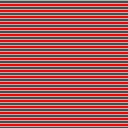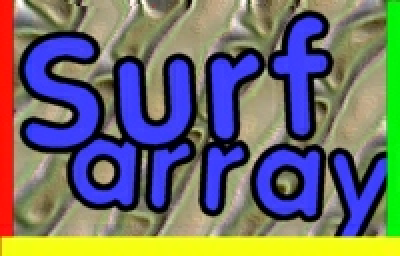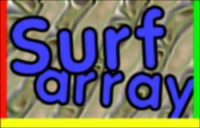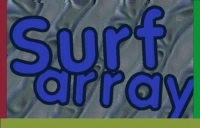## Surfarray Introduction¶

Author

Pete Shinners

Contact

pete@shinners.org

### Introduction¶

This tutorial will attempt to introduce users to both NumPy and the pygame surfarray module. To beginners, the code that uses surfarray can be quite intimidating. But actually there are only a few concepts to understand and you will be up and running. Using the surfarray module, it becomes possible to perform pixel level operations from straight python code. The performance can become quite close to the level of doing the code in C.

You may just want to jump down to the "Examples" section to get an idea of what is possible with this module, then start at the beginning here to work your way up.

Now I won't try to fool you into thinking everything is very easy. To get more advanced effects by modifying pixel values is very tricky. Just mastering Numeric Python (SciPy's original array package was Numeric, the predecessor of NumPy) takes a lot of learning. In this tutorial I'll be sticking with the basics and using a lot of examples in an attempt to plant seeds of wisdom. After finishing the tutorial you should have a basic handle on how the surfarray works.

### Numeric Python¶

If you do not have the python NumPy package installed, you will need to do that now. You can download the package from the NumPy Downloads Page To make sure NumPy is working for you, you should get something like this from the interactive python prompt.

```>>> from numpy import *                    #import numeric
>>> a = array((1,2,3,4,5))                 #create an array
>>> a                                      #display the array
array([1, 2, 3, 4, 5])
>>> a                                   #index into the array
3
>>> a*2                                    #new array with twiced values
array([ 2,  4,  6,  8, 10])
```

As you can see, the NumPy module gives us a new data type, the array. This object holds an array of fixed size, and all values inside are of the same type. The arrays can also be multidimensional, which is how we will use them with images. There's a bit more to it than this, but it is enough to get us started.

If you look at the last command above, you'll see that mathematical operations on NumPy arrays apply to all values in the array. This is called "element-wise operations". These arrays can also be sliced like normal lists. The slicing syntax is the same as used on standard python objects. (so study up if you need to :] ). Here are some more examples of working with arrays.

```>>> len(a)                                 #get array size
5
>>> a[2:]                                  #elements 2 and up
array([3, 4, 5])
>>> a[:-2]                                 #all except last 2
array([1, 2, 3])
>>> a[2:] + a[:-2]                         #add first and last
array([4, 6, 8])
>>> array((1,2,3)) + array((3,4))          #add arrays of wrong sizes
Traceback (most recent call last):
File "<stdin>", line 1, in <module>
ValueError: operands could not be broadcast together with shapes (3,) (2,)
```

We get an error on the last commend, because we try add together two arrays that are different sizes. In order for two arrays two operate with each other, including comparisons and assignment, they must have the same dimensions. It is very important to know that the new arrays created from slicing the original all reference the same values. So changing the values in a slice also changes the original values. It is important how this is done.

```>>> a                                      #show our starting array
array([1, 2, 3, 4, 5])
>>> aa = a[1:3]                            #slice middle 2 elements
>>> aa                                     #show the slice
array([2, 3])
>>> aa = 13                             #chance value in slice
>>> a                                      #show change in original
array([ 1, 2, 13,  4,  5])
>>> aaa = array(a)                         #make copy of array
>>> aaa                                    #show copy
array([ 1, 2, 13,  4,  5])
>>> aaa[1:4] = 0                           #set middle values to 0
>>> aaa                                    #show copy
array([1, 0, 0, 0, 5])
>>> a                                      #show original again
array([ 1, 2, 13,  4,  5])
```

Now we will look at small arrays with two dimensions. Don't be too worried, getting started it is the same as having a two dimensional tuple (a tuple inside a tuple). Let's get started with two dimensional arrays.

```>>> row1 = (1,2,3)                         #create a tuple of vals
>>> row2 = (3,4,5)                         #another tuple
>>> (row1,row2)                            #show as a 2D tuple
((1, 2, 3), (3, 4, 5))
>>> b = array((row1, row2))                #create a 2D array
>>> b                                      #show the array
array([[1, 2, 3],
[3, 4, 5]])
>>> array(((1,2),(3,4),(5,6)))             #show a new 2D array
array([[1, 2],
[3, 4],
[5, 6]])
```

Now with this two dimensional array (from now on as "2D") we can index specific values and do slicing on both dimensions. Simply using a comma to separate the indices allows us to lookup/slice in multiple dimensions. Just using "`:`" as an index (or not supplying enough indices) gives us all the values in that dimension. Let's see how this works.

```>>> b                                      #show our array from above
array([[1, 2, 3],
[3, 4, 5]])
>>> b[0,1]                                 #index a single value
2
>>> b[1,:]                                 #slice second row
array([3, 4, 5])
>>> b                                   #slice second row (same as above)
array([3, 4, 5])
>>> b[:,2]                                 #slice last column
array([3, 5])
>>> b[:,:2]                                #slice into a 2x2 array
array([[1, 2],
[3, 4]])
```

Ok, stay with me here, this is about as hard as it gets. When using NumPy there is one more feature to slicing. Slicing arrays also allow you to specify a slice increment. The syntax for a slice with increment is `start_index : end_index : increment`.

```>>> c = arange(10)                         #like range, but makes an array
>>> c                                      #show the array
array([0, 1, 2, 3, 4, 5, 6, 7, 8, 9])
>>> c[1:6:2]                               #slice odd values from 1 to 6
array([1, 3, 5])
>>> c[4::4]                                #slice every 4th val starting at 4
array([4, 8])
>>> c[8:1:-1]                              #slice 1 to 8, reversed
array([8, 7, 6, 5, 4, 3, 2])
```

Well that is it. There's enough information there to get you started using NumPy with the surfarray module. There's certainly a lot more to NumPy, but this is only an introduction. Besides, we want to get on to the fun stuff, correct?

### Import Surfarray¶

In order to use the surfarray module we need to import it. Since both surfarray and NumPy are optional components for pygame, it is nice to make sure they import correctly before using them. In these examples I'm going to import NumPy into a variable named N. This will let you know which functions I'm using are from the NumPy package. (and is a lot shorter than typing NumPy before each function)

```try:
import numpy as N
import pygame.surfarray as surfarray
except ImportError:
raise ImportError, "NumPy and Surfarray are required."
```

### Surfarray Introduction¶

There are two main types of functions in surfarray. One set of functions for creating an array that is a copy of a surface pixel data. The other functions create a referenced copy of the array pixel data, so that changes to the array directly affect the original surface. There are other functions that allow you to access any per-pixel alpha values as arrays along with a few other helpful functions. We will look at these other functions later on.

When working with these surface arrays, there are two ways of representing the pixel values. First, they can be represented as mapped integers. This type of array is a simple 2D array with a single integer representing the surface's mapped color value. This type of array is good for moving parts of an image around. The other type of array uses three RGB values to represent each pixel color. This type of array makes it extremely simple to do types of effects that change the color of each pixel. This type of array is also a little trickier to deal with, since it is essentially a 3D numeric array. Still, once you get your mind into the right mode, it is not much harder than using the normal 2D arrays.

The NumPy module uses a machine's natural number types to represent the data values, so a NumPy array can consist of integers that are 8-bits, 16-bits, and 32-bits. (the arrays can also use other types like floats and doubles, but for our image manipulation we mainly need to worry about the integer types). Because of this limitation of integer sizes, you must take a little extra care that the type of arrays that reference pixel data can be properly mapped to a proper type of data. The functions create these arrays from surfaces are:

`surfarray.``pixels2d`(surface)

Creates a 2D array (integer pixel values) that reference the original surface data. This will work for all surface formats except 24-bit.

`surfarray.``array2d`(surface)

Creates a 2D array (integer pixel values) that is copied from any type of surface.

`surfarray.``pixels3d`(surface)

Creates a 3D array (RGB pixel values) that reference the original surface data. This will only work on 24-bit and 32-bit surfaces that have RGB or BGR formatting.

`surfarray.``array3d`(surface)

Creates a 3D array (RGB pixel values) that is copied from any type of surface.

Here is a small chart that might better illustrate what types of functions should be used on which surfaces. As you can see, both the arrayXD functions will work with any type of surface.

32-bit

24-bit

16-bit

8-bit(c-map)

pixel2d

yes

yes

yes

array2d

yes

yes

yes

yes

pixel3d

yes

yes

array3d

yes

yes

yes

yes

### Examples¶

With this information, we are equipped to start trying things with surface arrays. The following are short little demonstrations that create a NumPy array and display them in pygame. These different tests are found in the arraydemo.py example. There is a simple function named surfdemo_show that displays an array on the screen.```allblack = N.zeros((128, 128))
surfdemo_show(allblack, 'allblack')
```

Our first example creates an all black array. Whenever you need to create a new numeric array of a specific size, it is best to use the `zeros` function. Here we create a 2D array of all zeros and display it.```striped = N.zeros((128, 128, 3))
striped[:] = (255, 0, 0)
striped[:,::3] = (0, 255, 255)
surfdemo_show(striped, 'striped')
```

Here we are dealing with a 3D array. We start by creating an all red image. Then we slice out every third row and assign it to a blue/green color. As you can see, we can treat the 3D arrays almost exactly the same as 2D arrays, just be sure to assign them 3 values instead of a single mapped integer.```imgsurface = pygame.image.load('surfarray.png')
rgbarray = surfarray.array3d(imgsurface)
surfdemo_show(rgbarray, 'rgbarray')
```

Here we load an image with the image module, then convert it to a 3D array of integer RGB color elements. An RGB copy of a surface always has the colors arranged as a[r,c,0] for the red component, a[r,c,1] for the green component, and a[r,c,2] for blue. This can then be used without caring how the pixels of the actual surface are configured, unlike a 2D array which is a copy of the `mapped` (raw) surface pixels. We will use this image in the rest of the samples.```flipped = rgbarray[:,::-1]
surfdemo_show(flipped, 'flipped')
```

Here we flip the image vertically. All we need to do is take the original image array and slice it using a negative increment.```scaledown = rgbarray[::2,::2]
surfdemo_show(scaledown, 'scaledown')
```

Based on the last example, scaling an image down is pretty logical. We just slice out all the pixels using an increment of 2 vertically and horizontally.```shape = rgbarray.shape
scaleup = N.zeros((shape*2, shape*2, shape))
scaleup[::2,::2,:] = rgbarray
scaleup[1::2,::2,:] = rgbarray
scaleup[:,1::2] = scaleup[:,::2]
surfdemo_show(scaleup, 'scaleup')
```

Scaling the image up is a little more work, but is similar to the previous scaling down, we do it all with slicing. First we create an array that is double the size of our original. First we copy the original array into every other pixel of the new array. Then we do it again for every other pixel doing the odd columns. At this point we have the image scaled properly going across, but every other row is black, so we simply need to copy each row to the one underneath it. Then we have an image doubled in size.```redimg = N.array(rgbarray)
redimg[:,:,1:] = 0
surfdemo_show(redimg, 'redimg')
```

Now we are using 3D arrays to change the colors. Here we set all the values in green and blue to zero. This leaves us with just the red channel.```factor = N.array((8,), N.int32)
soften = N.array(rgbarray, N.int32)
soften[1:,:]  += rgbarray[:-1,:] * factor
soften[:-1,:] += rgbarray[1:,:] * factor
soften[:,1:]  += rgbarray[:,:-1] * factor
soften[:,:-1] += rgbarray[:,1:] * factor
soften //= 33
surfdemo_show(soften, 'soften')
```

Here we perform a 3x3 convolution filter that will soften our image. It looks like a lot of steps here, but what we are doing is shifting the image 1 pixel in each direction and adding them all together (with some multiplication for weighting). Then average all the values. It's no Gaussian, but it's fast. One point with NumPy arrays, the precision of arithmetic operations is determined by the array with the largest data type. So if factor was not declared as a 1 element array of type numpy.int32, the multiplications would be performed using numpy.int8, the 8 bit integer type of each rgbarray element. This will cause value truncation. The soften array must also be declared to have a larger integer size than rgbarray to avoid truncation.```src = N.array(rgbarray)
dest = N.zeros(rgbarray.shape)
dest[:] = 20, 50, 100
diff = (dest - src) * 0.50
```

Lastly, we are cross fading between the original image and a solid bluish image. Not exciting, but the dest image could be anything, and changing the 0.50 multiplier will let you choose any step in a linear crossfade between two images.

Hopefully by this point you are starting to see how surfarray can be used to perform special effects and transformations that are only possible at the pixel level. At the very least, you can use the surfarray to do a lot of Surface.set_at() Surface.get_at() type operations very quickly. But don't think you are finished yet, there is still much to learn.

### Surface Locking¶

Like the rest of pygame, surfarray will lock any Surfaces it needs to automatically when accessing pixel data. There is one extra thing to be aware of though. When creating the pixel arrays, the original surface will be locked during the lifetime of that pixel array. This is important to remember. Be sure to "del" the pixel array or let it go out of scope (ie, when the function returns, etc).

Also be aware that you really don't want to be doing much (if any) direct pixel access on hardware surfaces (HWSURFACE). This is because the actual surface data lives on the graphics card, and transferring pixel changes over the PCI/AGP bus is not fast.

### Transparency¶

The surfarray module has several methods for accessing a Surface's alpha/colorkey values. None of the alpha functions are affected by overall transparency of a Surface, just the pixel alpha values. Here's the list of those functions.

`surfarray.``pixels_alpha`(surface)

Creates a 2D array (integer pixel values) that references the original surface alpha data. This will only work on 32-bit images with an 8-bit alpha component.

`surfarray.``array_alpha`(surface)

Creates a 2D array (integer pixel values) that is copied from any type of surface. If the surface has no alpha values, the array will be fully opaque values (255).

`surfarray.``array_colorkey`(surface)

Creates a 2D array (integer pixel values) that is set to transparent (0) wherever that pixel color matches the Surface colorkey.

### Other Surfarray Functions¶

There are only a few other functions available in surfarray. You can get a better list with more documentation on the `surfarray reference page`. There is one very useful function though.

`surfarray.``blit_array`(surface, array)

This will transfer any type of 2D or 3D surface array onto a Surface of the same dimensions. This surfarray blit will generally be faster than assigning an array to a referenced pixel array. Still, it should not be as fast as normal Surface blitting, since those are very optimized.

There's a couple last things you should know about NumPy arrays. When dealing with very large arrays, like the kind that are 640x480 big, there are some extra things you should be careful about. Mainly, while using the operators like + and * on the arrays makes them easy to use, it is also very expensive on big arrays. These operators must make new temporary copies of the array, that are then usually copied into another array. This can get very time consuming. Fortunately, all the NumPy operators come with special functions that can perform the operation "in place". For example, you would want to replace `screen[:] = screen + brightmap` with the much faster `add(screen, brightmap, screen)`. Anyway, you'll want to read up on the NumPy UFunc documentation for more about this. It is important when dealing with the arrays.

Another thing to be aware of when working with NumPy arrays is the datatype of the array. Some of the arrays (especially the mapped pixel type) often return arrays with an unsigned 8-bit value. These arrays will easily overflow if you are not careful. NumPy will use the same coercion that you find in C programs, so mixing an operation with 8-bit numbers and 32-bit numbers will give a result as 32-bit numbers. You can convert the datatype of an array, but definitely be aware of what types of arrays you have, if NumPy gets in a situation where precision would be ruined, it will raise an exception.

Lastly, be aware that when assigning values into the 3D arrays, they must be between 0 and 255, or you will get some undefined truncating.